# 拉普拉斯锐化图像

拉普拉斯锐化图像是根据图像某个像素的周围像素到此像素的突变程度有关，也就是说它的依据是图像像素的变化程度。我们知道，一个函数的一阶微分描述了函数图像是朝哪里变化的，即增长或者降低；而二阶微分描述的则是图像变化的速度，急剧增长下降还是平缓的增长下降。那么据此我们可以猜测出依据二阶微分能够找到图像的色素的过渡程度，例如白色到黑色的过渡就是比较急剧的。

或者用官方点的话说：当邻域中心像素灰度低于它所在的领域内其它像素的平均灰度时，此中心像素的灰度应被进一步降低，当邻域中心像素灰度高于它所在的邻域内其它像素的平均灰度时，此中心像素的灰度应被进一步提高，以此实现图像的锐化处理。

运用拉普拉斯可以增强图像的细节，找到图像的边缘。但是有时候会把噪音也给增强了，那么可以在锐化前对图像进行平滑处理。

先看一阶偏微分和推出的二元函数微分：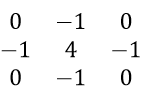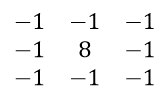【注】从上面的两种模板中就可以看出，如果一个黑色平面中有一个白点，那么模板矩阵可以使这个白点更亮。由于图像边缘就是灰度发生跳变的区域，所以拉普拉斯模板对边缘检测很有用。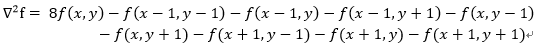%拉普拉斯算子锐化图像，用二阶微分
%四邻接g(x,y)=[f(x+1,y)+f(x-1,y)+f(x,y+1)+f(x,y-1)]-4f(x,y)
clear
clc
I1=imread('D:\BingZhouWork\Image\lena.jpg');
I=im2double(I1);
[m,n,c]=size(I);
A=zeros(m,n,c);
%分别处理R、G、B
%先对R进行处理
for i=2:m-1
for j=2:n-1
A(i,j,1)=I(i+1,j,1)+I(i-1,j,1)+I(i,j+1,1)+I(i,j-1,1)-4*I(i,j,1);
end
end

%再对G进行处理
for i=2:m-1
for j=2:n-1
A(i,j,2)=I(i+1,j,2)+I(i-1,j,2)+I(i,j+1,2)+I(i,j-1,2)-4*I(i,j,2);
end
end

%最后对B进行处理
for i=2:m-1
for j=2:n-1
A(i,j,3)=I(i+1,j,3)+I(i-1,j,3)+I(i,j+1,3)+I(i,j-1,3)-4*I(i,j,3);
end
end
B=I-A;

imwrite(B,'lena.tif','tif');
imshow('D:\BingZhouWork\Image\lena.jpg');title('不清晰图像');figure
imshow('lena.tif');title('得到的清晰图像')

%matlab直接调用拉普拉斯方法
clear
clc
f=imread('D:\BingZhouWork\Image\unclear.jpg');
f2=im2double(f); %将f转换归一化的double类图像，然后进行滤波
w=fspecial('laplacian',0);
g1=imfilter(f,w,'replicate');
g=f2-g1;
imshow(f);figure
imshow(g);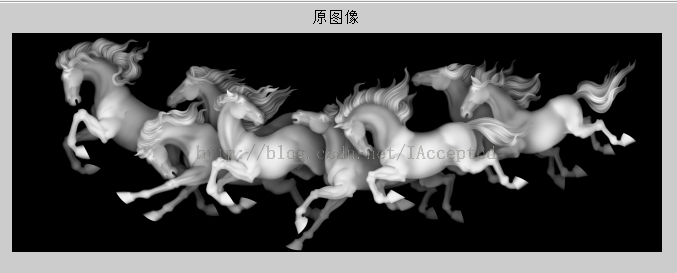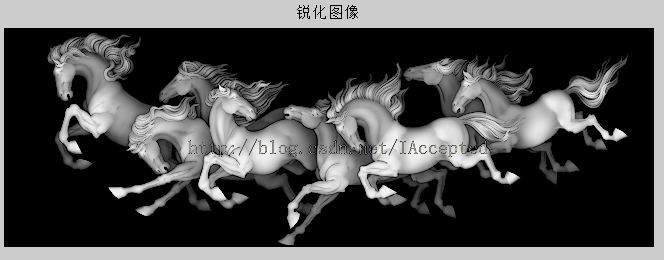06-1407-13
06-07
12-02
09-07
04-157996
09-042万+
03-141555
10-11988
07-222598
12-111万+
07-27180
04-231万+
03-204053
©️2020 CSDN 皮肤主题: 技术黑板 设计师:CSDN官方博客